ccComboPoints align - WoWInterface
 08-11-08, 12:21 PM #1 Imithat A Fallenroot Satyr Join Date: May 2008 Posts: 25ccComboPoints align Hey guys, I need a little bit help with the addon ccComboPoints ( Found here!) The mod display the combopoints in a very cool look in center of the screen! But how i can change this? I would it a little bit higher of my action bar. i changed the cords in the ccComboPoints.lua on the follow line (but it doesn't work): Code: ```local CPLayouts = {  = {  = {["x"] = -58, ["y"] = -148+32 },  = {["x"] = -30, ["y"] = -160+32 },  = {["x"] = 0, ["y"] = -166+32 },  = {["x"] = 30, ["y"] = -160+32 },  = {["x"] = 58, ["y"] = -148+32 } },  = {  = {["x"] = -58, ["y"] = 148-150 },  = {["x"] = -30, ["y"] = 160-150 },  = {["x"] = 0, ["y"] = 166-150 },  = {["x"] = 30, ["y"] = 160-150 },  = {["x"] = 58, ["y"] = 148-150 } } };``` pls help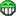__________________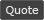WoWInterface » ccComboPoints align

 Thread Tools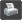Show Printable Version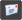Email this Page Display Modes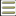Linear Mode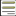Switch to Hybrid Mode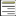Switch to Threaded Mode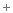Posting Rules You may not post new threads You may not post replies You may not post attachments You may not edit your posts vB code is On Smilies are On [IMG] code is On HTML code is Off